Related Articles

# Class 8 NCERT Solutions – Chapter 4 Practical Geometry – Exercise 4.5

• Last Updated : 20 Nov, 2020

### 1. The square READ with RE = 5.1 cm.

Steps of construction:

Step 1: Draw one side of the square RE = 5.1 cm.

Step 2: From E draw an angle of 90°.

Step 3: From E cut EA of 5.1 cm.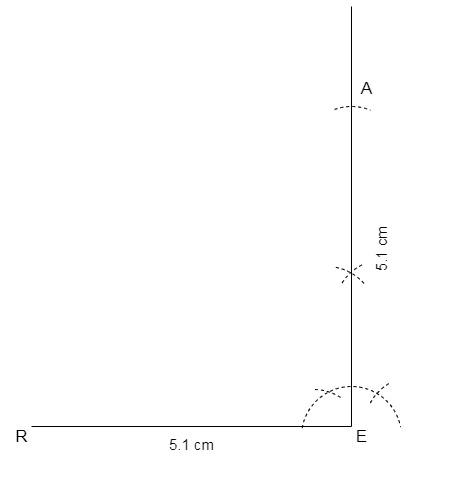Step 4: Now draw an arc of 5.1 cm from both A and R, intersect them and mark the intersection point as D.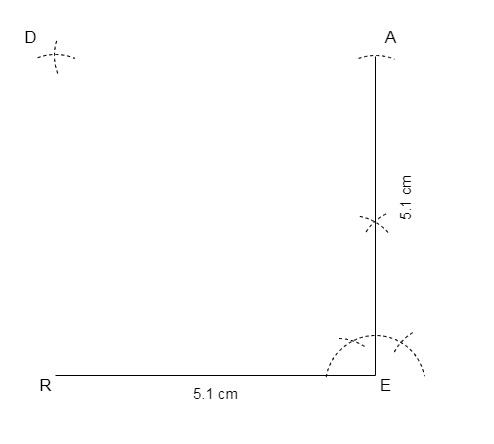Step 5: Join RD and ED.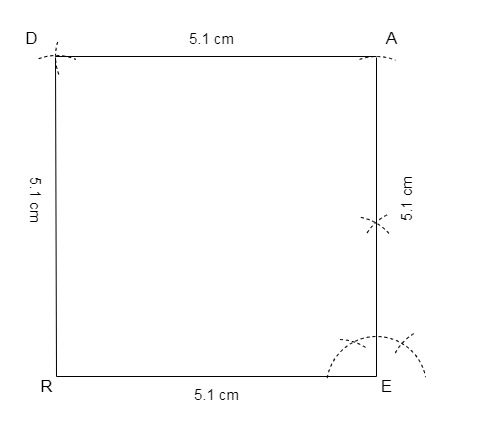Thus, we have the required square READ.

### 2. A rhombus whose diagonals are 5.2 cm and 6.4 cm long.

Steps of construction:

Let the rhombus be ABCD with diagonals, AC = 5.2 cm and BD = 6.4 cm.

Step 1: Draw a line AC of 5.2 cm

Step 2: Draw perpendicular bisector of AC and mark the bisector point as O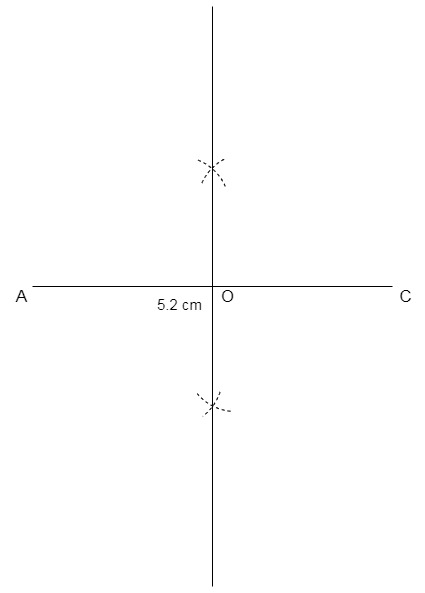Step 3: Draw two arcs with centre O of radius 1/2 × BD = 1/2 × 6.4 cm = 3.2 cm and these arcs will meet the bisector at point B and D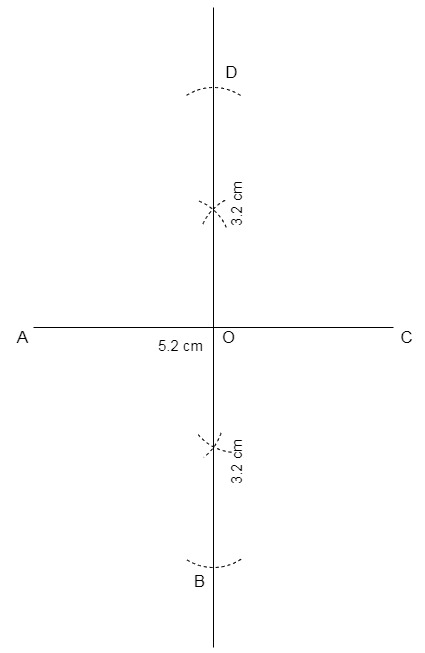Step 4: Join AB, BC, CD and AD.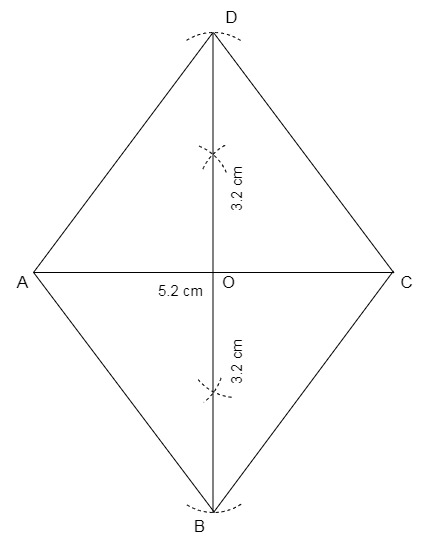Hence, we have the required rhombus ABCD.

### 3. A rectangle with adjacent sides of lengths 5 cm and 4 cm.

Steps of construction:

Step 1: Let the rectangle be CODE with adjacent sides CO = 5 cm and OD = 4 cm.

Step 2: Draw CO = 5 cm.

Step 3: From O draw an angle of 90°.

Step 4: From O cut OD = 4 cm.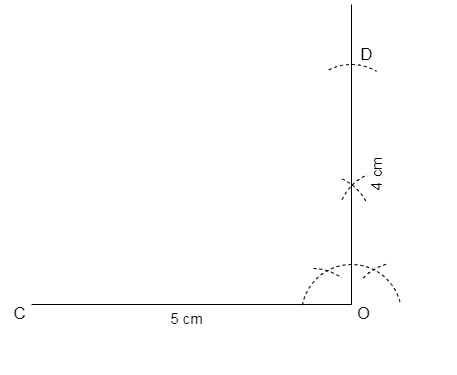Step 5: From C draw an arc of 4 cm and from D draw an arc of 5 cm, intersect both the arcs and mark the point as E.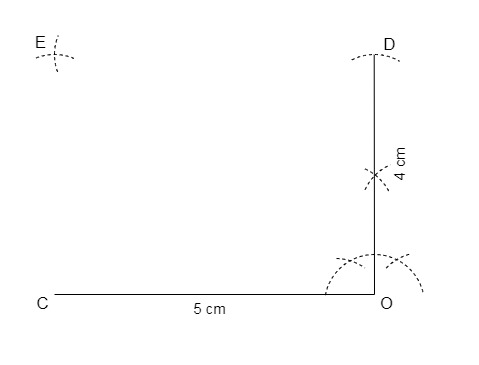Step 6: Join CE and DE.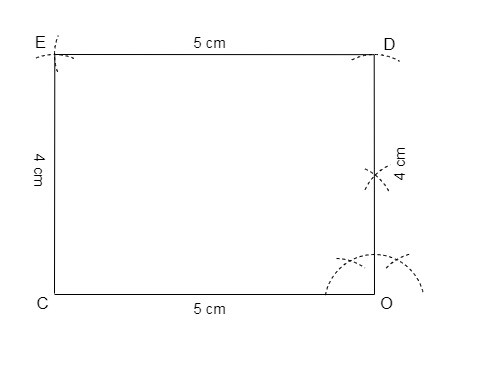Thus, we have the required rectangle CODE.

### 4. A parallelogram OKAY where OK = 5.5 cm and KA = 4.2 cm. Is it unique?

Steps of construction:

Step 1: Draw a line OK of 5.5 cm.

Step 2: Draw a ray at K at any convenient angle, let the angle be 60°.

Step 3: Now cut the ray at 4.2 cm and mark the point as A.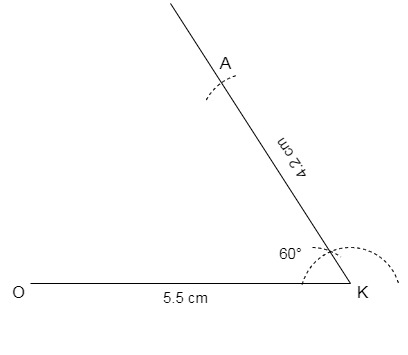Step 4: From A draw an arc of 5.5 cm and from O draw an arc of 4.2 cm, intersect both the arcs at Y.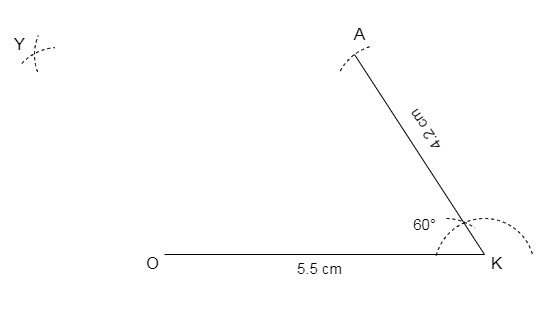Step 5: Join OY and AY.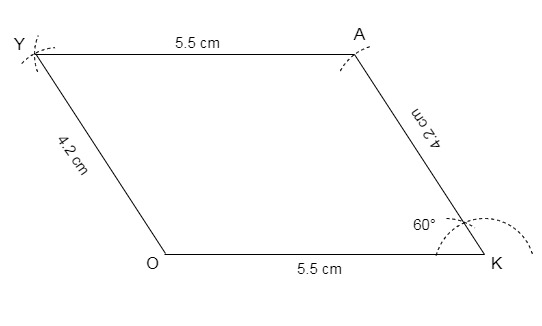Thus, we have the required parallelogram OKAY.
No, it is not unique as angle K can be any angle.

Attention reader! Don’t stop learning now. Join the First-Step-to-DSA Course for Class 9 to 12 students , specifically designed to introduce data structures and algorithms to the class 9 to 12 students

My Personal Notes arrow_drop_up